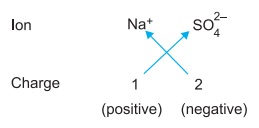# Chemical Formula of Compounds

A compound is made of two or more than two elements combined in a definite proportion by mass (law of constant proportions). Thus, the number of combining atoms in a compound is fixed. The elements are represented by their symbols (H for hydrogen, Na for sodium).

Similarly a compound is also represented by a shorthand notation known as formula or chemical formula. The formula of a compound indicates

1. Elements constituting the compound
2. The number of each constituent element

In other word, the formula of a compound also represents its chemical composition. The atoms of elements constituting a compound are indicated by their symbols and their number is indicated as a subscript on the right hand bottom of the symbol. For example, in the formula of water, H2O, two atoms of hydrogen are indicated as subscript "2", while oxygen is shown without writing any subscript, which means that the number of oxygen atom is just one.

### Valency and Formulation

Every element has a definite capacity to combine with other elements. This combining capacity of an element is called its valency. Valency depends on the electronic configuration of elements.

Most of the simple compounds are made of two elements. Such compounds are called binary compounds. It is easy to write formula of such compounds. When a metal combines with a non-metal, the symbol of the metal-element is written on the left hand side and that of the non-metal element on right hand side.

If both are non-metal, we write more electronegative element on the right hand side. In naming a compound, the first element is written as such and the name of the second element (more electronegative element) changes its ending to "ide". For writing chemical formula, you have to write valencies and then cross over the valencies of the combining atoms. Formula of the compounds resulting from carbon and chlorine, hydrogen and oxygen, and hydrogen and chlorine can be written as:Thus, you can write formulas of various compounds if you know elements constituting them and their valencies.

Valency depends on the electronic configuration and nature of the elements. Sometimes an element shows more than one type of valency. These elements have variable valency. For example nitrogen forms several oxides: N2O, N2O2, N2O3, N2O4 and N2O5. If you take valency of oxygen equal to 2, then valency of nitrogen in the oxides will be 1, 2, 3, 4 and 5 respectively.

Valencies are not always fixed. Similar to nitrogen, phosphorus also shows valencies 3 and 5 as reflected in compounds PBr3 and P2O5. In these compounds, there are more than one atom. In such cases, number of atoms is indicated by attaching a numerical prefix (mono, di, tri).

Numerical Prefixes

1. Mono: carbon monoxide, CO
2. Di: carbon dioxide, CO2
3. Tri: phosphorus trichloride, PCl3
4. Tetra: carbon tetrachloride, CCl4
5. Penta: Dinitrogen pentoxide, N2O5

The "–o" or "–a" at the end of the prefix is often dropped before another vowel. For example, monoxide, pentoxide. There is no gap between numerical prefix and the name of the element. The prefix mono is usually dropped for the first element. When hydrogen is the first element in the formula, no prefix is added before hydrogen irrespective of the number. For example, the compound H2S is named as hydrogen sulphide and not as dihydrogen sulphide.

Thus, writing formula of a binary compound is relatively easy. However, when you have to write formula of a compound which involves more than two elements (polyatomic molecule), it is somewhat a cumbersome task.

There are basically two types of compounds: covalent compounds and ionic or electrovalent compounds. H2O and NH3 are covalent compounds. NaCl and MgO are ionic compounds. An ionic compound is made of two charged constituents. One positively charged and other negatively charged. In case of NaCl, there are two ions : Na+ and Cl ion. Charge of these ions in case of electrovalent compound is used for writing formula.

It is easy to write formula of an ionic compound only if there is one metal and one non-metal as in the case of NaCl and MgO. If there are more than two elements in an ionic compound, formulation will be a little difficult and in that situation you should know charge of cations and anions.

### Formulation of Ionic Compounds

Formulation of an ionic compound is easy when you know charge of cation and anion. In an ionic compound, sum of the charge of cation and anion should be equal to zero.

For example, suppose you have to write formula of sodium sulphate which is made of Na+ and SO42– ions. For this the positive and negative charge can be crossed over to give subscripts. The purpose of this crossing over of charges is to find the number of ions required to equate the number of positive and negative charges.This gives the formula of sodium sulphate as Na2SO4.